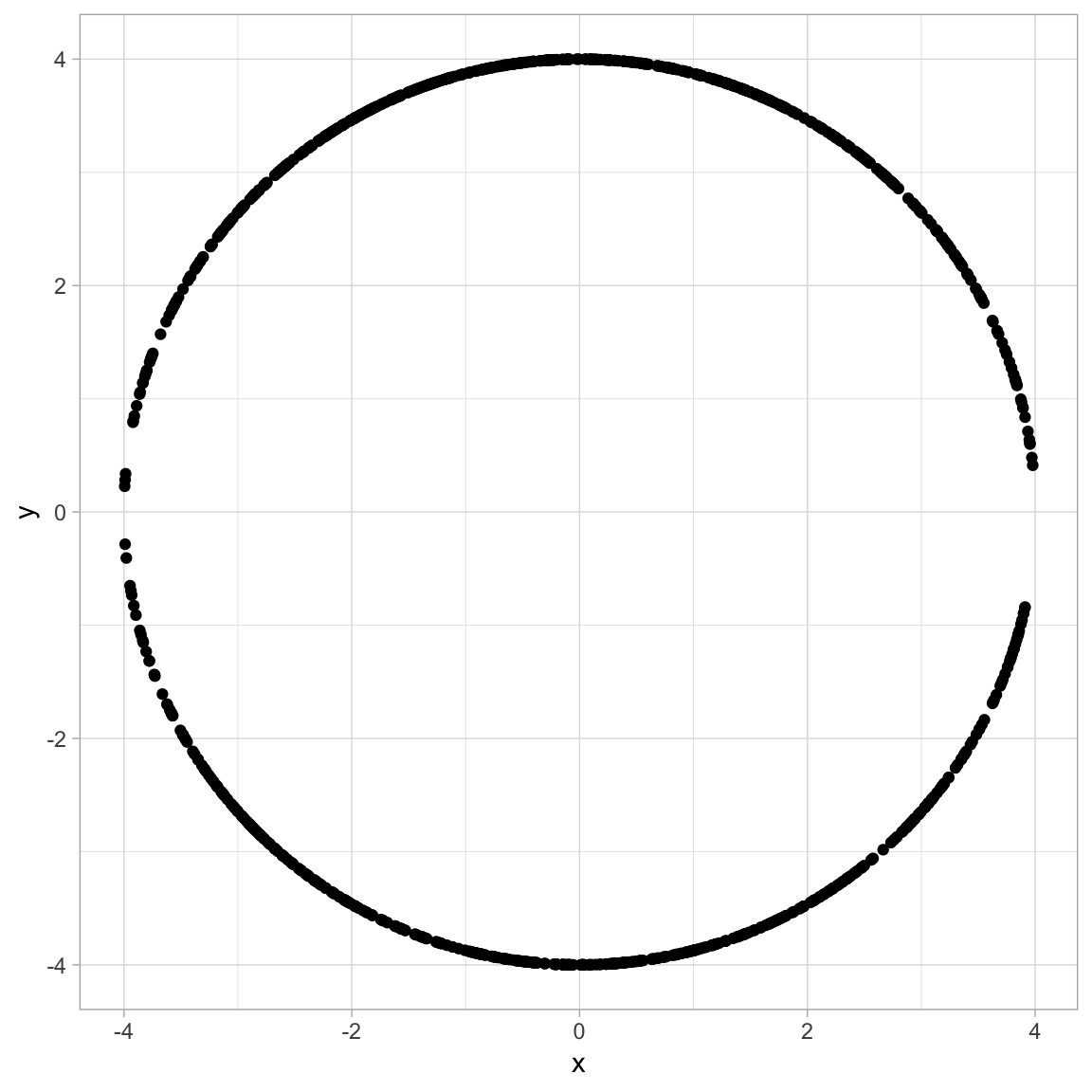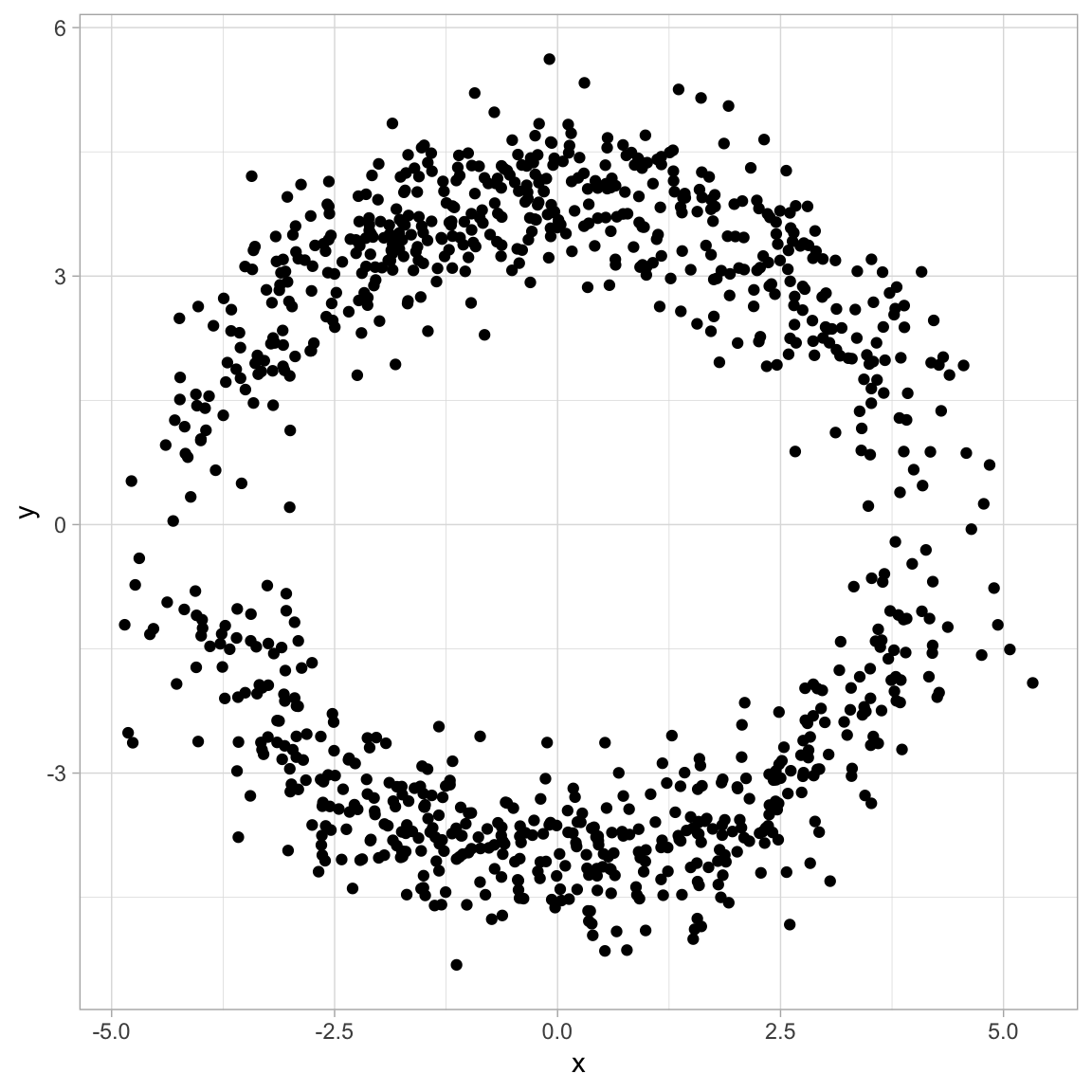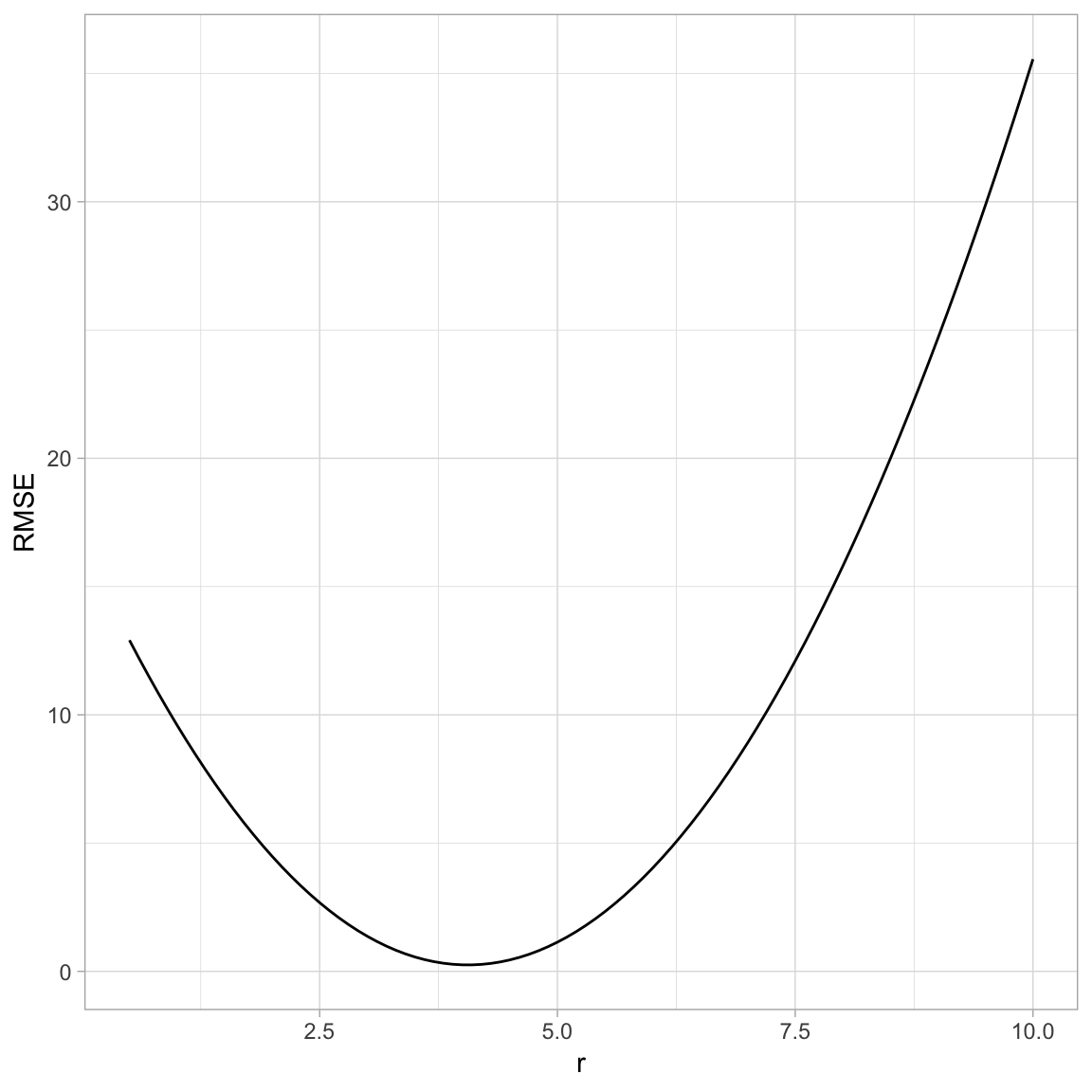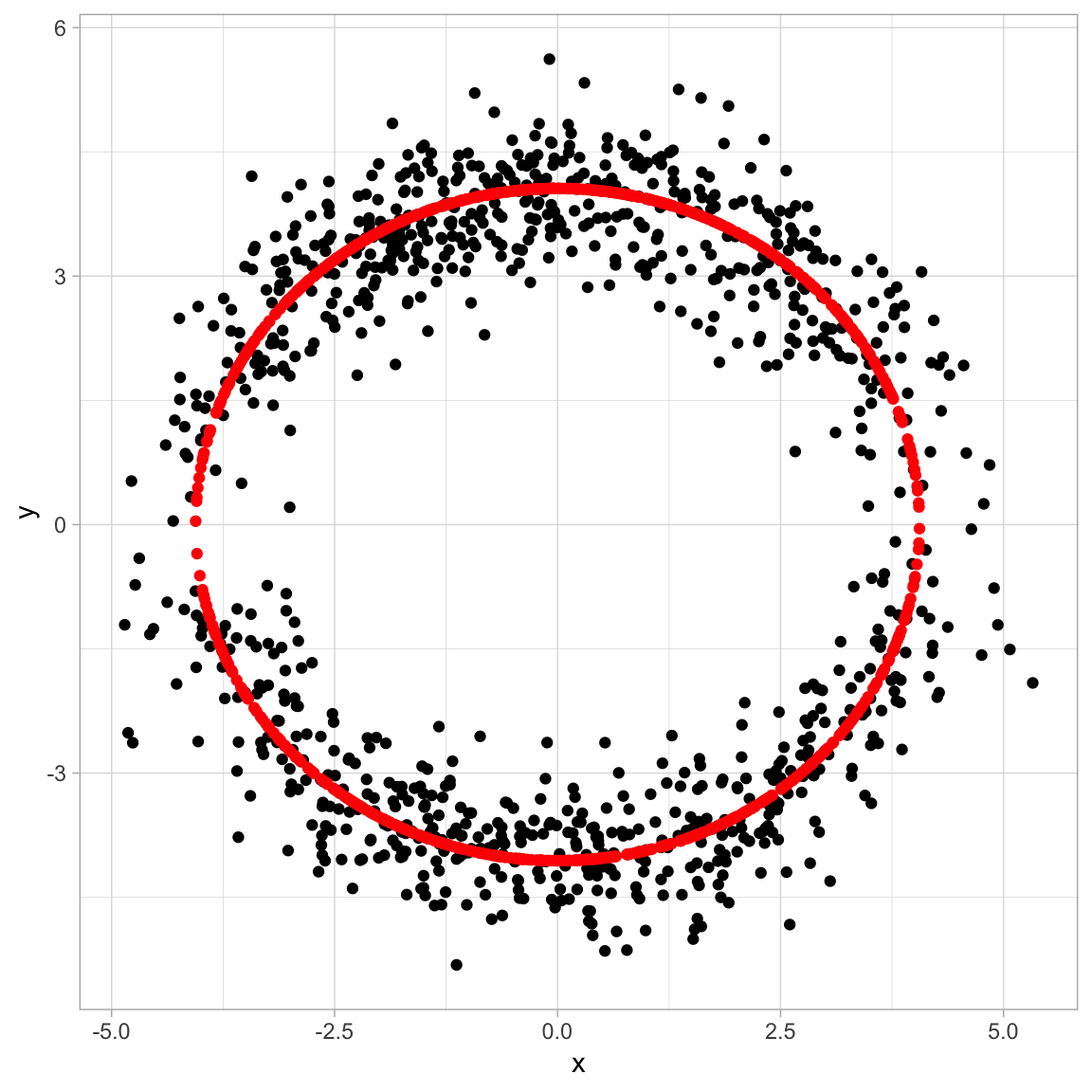# Circle Radius Fit for a Cloud of Points

In this post I explore how to render a .Rmd file directly with blogdown. To play around with it, I wrote a simple R notebook which fits a circle to a cloud of points.

# Prepare the Notebook

library(tidyverse)

# Generate Circle Data

# Dimension of the space.
d <- 2

# Number of sample points.
N <- 1000

R <- 4

# Generate random sample of points (x - axis).
points.0 <- 1:N %>% map(.f = ~ runif(n = d - 1, min =  - R, max = R))

# Generate the corresponding y - coordinates.
all.points <- points.0 %>% map(.f = ~ c(., sign(runif(n = 1, min = - 1, max = 1))*sqrt(R^2 - norm(., type = '2')^2)))

# Store data in a tibble.
all.points.df <- all.points %>% reduce(.f = ~ rbind(.x, .y)) %>%
as.tibble %>%
rename(x = V1, y = V2)

# Plot the data.
all.points.df %>%
ggplot() +
theme_light() +
geom_point(mapping = aes(x = x, y = y))We add noise from a normal disttribution with mean zero and standard deviation sd.

# Set standard deviation.
sd <- 0.5

all.samples <- all.points %>% map(.f =  ~ . + rnorm(n = d, mean = 0, sd = sd))

# Store data in a tibble.
all.samples.df <- all.samples %>% reduce(.f = ~ rbind(.x, .y)) %>%
as.tibble %>%
rename(x = V1, y = V2)

# Plot the data.
all.samples.df %>%
ggplot() +
theme_light() +
geom_point(mapping = aes(x = x, y = y))# Define Optimization Function

In order to find the best fitting circle, we need to define what “best” means. We aim to minimize the RMSE.

# Define function to optimize.
ComputeRMSE <- function(all.samples, r, N) {

all.samples %>% map_dbl(.f = ~ (r - norm(., type = '2'))^2) %>% mean

}

Let us visualize the shape of the cost function.

rmse.df <- seq(from = 0.5, to = 10, by = 0.1) %>%
map(.f = ~ c(., ComputeRMSE(all.samples = all.samples, r = ., N = N ))) %>%
reduce(.f = ~ rbind(.x, .y)) %>%
as.tibble %>%
rename(r = V1, RMSE = V2)

rmse.df %>%
ggplot() +
theme_light() +
geom_line(mapping = aes(x = r, y = RMSE))We aim to find the minimum.

# Run Optimization

opt.obj <- optimize(f = function(r) ComputeRMSE(all.samples = all.samples, r = r, N = N),
interval = 1:10)

r.hat <- opt.obj\$minimum

r.hat
##  4.057873

# Visualize Results

We project each sample point onto the best circle fit.

all.samples %>% map(.f = function(x) r.hat*x /norm(x, type = '2')) %>%
reduce(.f = function(x, y) rbind(x, y)) %>%
as.tibble %>%
rename(x1 = V1, y1 =V2) %>%
cbind(all.samples.df) %>%
ggplot() +
theme_light() +
geom_point(mapping = aes(x = x, y = y)) +
geom_point(mapping = aes(x = x1, y = y1), color = 'red')# Analytical Solution

By taking the derivative of the cost function with respect to r we can easily get the value of r.hat.

r.hat <- all.samples %>% map_dbl(.f = ~ norm(., type = '2')) %>% mean

r.hat
##  4.057873